Sunday 25th July 2021# Classroom Key Stage 2 / KS2 - Maths Activities & Resources

## Welcome to the Maths section of the Key Stage 2 classroom. Within KS2 Maths you will find online @school assessment tests that will help children to enhance their learning and revise the subject of Maths at Key Stage 2 level.

•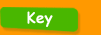•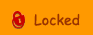••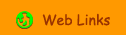•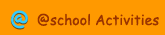•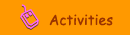•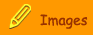###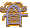Division

Divide by 10 - Interactive activity to learn how to multiply by 10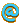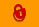Divide by 100 - Interactive activity to learn how to multiply by 10Divide by 1000 - Interactive activity to divide by 1000Divide by 4 - Interactive activity to use halving to divide by 4Dividing Decimals by 10 or 100 - Interactive activity to learn how to divide decimals by 10 or 100Division - Interactive activities for grouping and sharingDivision - Interactive activities about division from the BBCDivision - Year 5 - Interactive activity about using and applying division.Division Factory - Interactive activity about divisionDivision Info - Interactive information about division.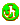Division Sums - Year 6 - Use this interactive activity about division using written methods or a calculator.Division with Remainders - Interactive activity to learn about remainders when dividingDivision with Remainders - Year 4 - Interactive activity about division with remainders.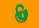Halving 2-Digit Numbers - Interactive activity to learn how to halve numbers with 2- digits.Halving 3-Digit Numbers - Interactive activity to learn how to halve numbers with 3 - digits.Maths Generator - Create online sums either online or as a worksheet including addition, subtraction, multiplication and division.Maths Square - 1 - Print and do activity about placing numbers into a number grid.Operations - Clipart of operators.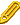Reverse Multiplication - Interactive activity to use the relationship between multiplication and divisionSharing Cakes - Interactive division activitySum Sense - Division - Interactive activity about divisionYear 5 Division  Remainders - Printable activity to give a remainder as a fractionYear 5 Remainders - Printable and interactive activities about remainders after division